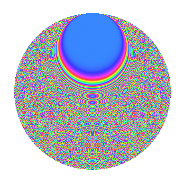# Properties

 Label 2015.2.etLevel 2015 Weight 2 Character orbit et Rep. character $$\chi_{2015}(47,\cdot)$$ Character field $$\Q(\zeta_{20})$$ Dimension 1760 Sturm bound 448

# Related objects

## Defining parameters

 Level: $$N$$ = $$2015 = 5 \cdot 13 \cdot 31$$ Weight: $$k$$ = $$2$$ Character orbit: $$[\chi]$$ = 2015.et (of order $$20$$ and degree $$8$$) Character conductor: $$\operatorname{cond}(\chi)$$ = $$2015$$ Character field: $$\Q(\zeta_{20})$$ Sturm bound: $$448$$

## Dimensions

The following table gives the dimensions of various subspaces of $$M_{2}(2015, [\chi])$$.

Total New Old
Modular forms 1824 1824 0
Cusp forms 1760 1760 0
Eisenstein series 64 64 0

## Trace form

 $$1760q - 12q^{3} + 432q^{4} - 16q^{5} - 32q^{6} - 12q^{7} + O(q^{10})$$ $$1760q - 12q^{3} + 432q^{4} - 16q^{5} - 32q^{6} - 12q^{7} + 24q^{10} - 12q^{11} + 24q^{12} - 6q^{13} - 24q^{15} - 440q^{16} - 64q^{18} + 16q^{19} - 68q^{20} - 36q^{21} + 48q^{22} + 8q^{23} - 40q^{24} - 8q^{25} - 32q^{26} + 4q^{28} + 32q^{30} - 32q^{31} - 16q^{34} + 12q^{35} - 8q^{37} - 16q^{38} + 16q^{39} + 20q^{40} - 20q^{41} - 60q^{42} - 8q^{43} - 40q^{44} + 32q^{45} + 12q^{46} - 76q^{47} + 44q^{48} - 392q^{49} - 60q^{50} - 32q^{52} - 24q^{53} + 8q^{54} + 12q^{55} - 28q^{58} + 24q^{59} + 76q^{60} - 64q^{61} + 40q^{62} + 432q^{64} - 26q^{65} - 40q^{66} - 16q^{69} + 122q^{70} - 60q^{71} + 28q^{72} - 56q^{75} + 116q^{76} + 128q^{77} - 144q^{78} - 136q^{80} + 384q^{81} - 16q^{82} - 56q^{83} + 24q^{85} - 28q^{86} - 104q^{87} - 196q^{90} - 20q^{91} - 32q^{92} - 8q^{95} - 52q^{96} - 24q^{99} + O(q^{100})$$

## Decomposition of $$S_{2}^{\mathrm{new}}(2015, [\chi])$$ into irreducible Hecke orbits

The newforms in this space have not yet been added to the LMFDB.